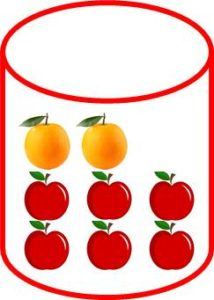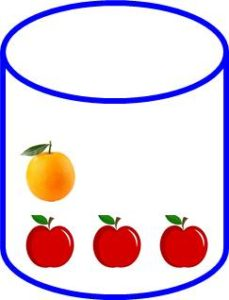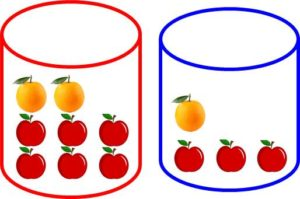# Machine Learning 101 – Introduction to Probability Theory

As you already know, one of the four basic theories of Machine Learning is the Probability Theory. Or simply, Probability.  And this is one challenge of Machine Learning. Big words are always used to describe simple concepts. But, we’ll handle it as we move. So don’t worry!

We’ll cover the following:

#### 1. Basics of Probability

We would proceed by taking some examples.

Assuming you have a red box, that contains 2 apples and 6 oranges. If you take one fruit from this box randomly:• what is the probability that it is an apple? 6/8 = 4/4 (that is number of apples divided by total number of fruits)
• what is the probability that it is an orange? 2/8 = 1/4 (that is number of oranges divided by total number of fruits.

Assuming you have a blue box containing 3 apples and one orange. If you randomly pick a fruit from this box:• what is the probability that it is an apple?
• what is the probability that it is an orange?

I’m sure you can do this. Leave your answer as a comment below. So I know you are following.

#### 2. Simple Example

What if we select a fruit from the red box and this fruit is an orange. Then we select another fruit from the same red box (without putting back the first one).

What is the probability that this second fruit is an apple?

Consider that the first was an orange. It then mean that that the total number of fruits is now 7. Therefore the probability that the second fruit is an apple is 6/7

What is the probability that it is an orange?

Similarly, only 7 fruits remain in the box. Moreover, an orange was removed the first time. So only one orange is left. Therefore, the probability that the second fruit is an orange is 1/7.

In this two cases, since we were given a prior condition, we can therefore call it conditional probability. We’ll discuss this more later.

#### 3. Experiments and Random Variables

In Machine Learning we often talk about experiments. This is same as used in statistics. This means that we carry out certain procedure, then at the end, we reach certain conclusion or make certain inference.

An random variable on the other hand is a variable can can take on random values.

So let’s take a simple experiment.  In this experiment, we put the two boxes side by side. Then we randomly choose one box. From this box, we also randomly select a fruit and record what fruit it is.

We do this 100 time and figure out that 60% of the time ( that is 6/10), we selected the blue box and 40% of the time, the red ball was selected( that is 4/10)Let B be a random variable whose value represents the particular box (whether red or blue). B can randomly have two possible values:  a value of r or b. r for red box and b for blue box.

Also, let F be a random variable that represents the fruits. F can randomly take two possible values: a or o. r a for apple and o for orange.

#### 4. Some Basic Notations

Let’s now write the outcome of the experiments using probability notations.

Based on the experiment, the probability of selecting the blue ball is 6/10. This can be written as:

P(B = b) = 6/10

This can be written is shorter form as:

P(b) = 6/10

Also, the probability of selecting the red box is 4/10 and can be written as:

P(B=r) = 4/10

This can be written in shorter form as:

P(r) = 4/10

Note: values of probabilities must always be between 0 and 1

We’ll stop here for now. Next, we’ll consider some laws of probability. But, I’ll like you  to solve the quiz below. It is based on the previous example.

#### 5. Exercises for You

a). Suppose select a fruit from the blue box. Then we replace it an select again from the same blue box.

• What is the probability that both fruits are apples.
• What is the probability that that first fruit is apple and the second is orange
• What is the probability that the both fruits are oranges.
• What is the probability that the first fruit is orange and the second is apple.

b). Solve the same four probabilities but this time suppose the first fruit was not replaced before the second selection.#### kindsonthegenius

Kindson Munonye is currently completing his doctoral program in Software Engineering in Budapest University of Technology and Economics

View all posts by kindsonthegenius →